Next: *GAP Up: Input deck format Previous: *FREQUENCY   Contents

## *FRICTION

Keyword type: model definition, surface interaction

With this option the friction behavior of a surface interaction can be defined. The friction behavior is optional for contact analyses. There are no parameters.

The frictional behavior defines the relationship between the shear stress in the contact area and the relative tangential displacement between the slave and the master surface. It is characterized by a linear range with tangent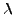(stick slope) for small relative displacements (stick) followed by a horizontal upper bound (slip) given by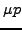, where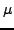is the friction coefficient and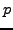the local pressure (Figure 119).is dimensionless and usually takes values between 0.1 and 0.5,has the dimension of force per volume and should be chosen to be about 10 times smaller than the spring constant.

For face-to-face penalty contact with PRESSURE-OVERCLOSURE=TIED the value of the friction coefficient is irrelevant.

First line:

• *FRICTION

Following line for all types of analysis except modal dynamics:

•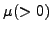.
•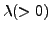.

Example:

*FRICTION
0.2,5000.


defines a friction coefficient of 0.2 and a stick slope of 5000.

Example files: friction1, friction2.Next: *GAP Up: Input deck format Previous: *FREQUENCY   Contents
guido dhondt 2014-03-02